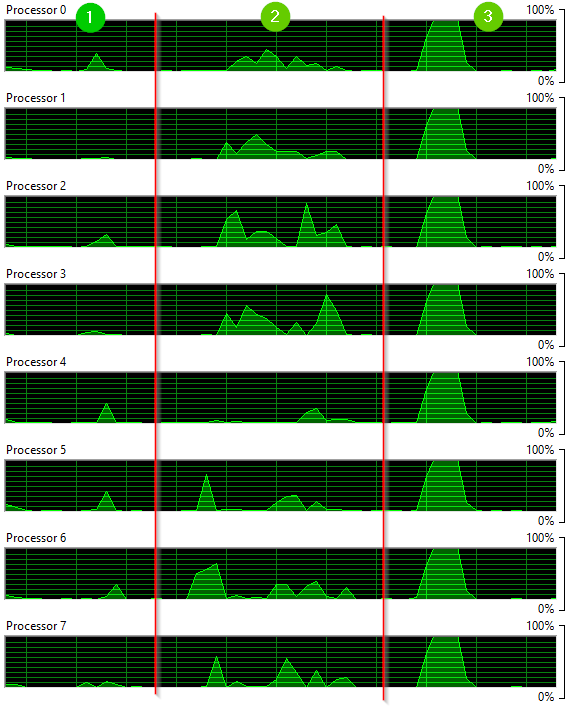Resharper tells us to convert for(each) loops to Linq. An argument I read was that Linq queries offer options for parallelization.

Iterate over each item in parallel, making it faster on hardware that offers more than one processor.

To put this to the test I made a quick experiment.

# The Problem

To simulate a problem, I want to compare two byte arrays and count how many items are the same.

``````int Compare(byte[] dataA, byte[] dataB)
``````

# Implementations

First, the naive approach:

``````static int CompareNaive(byte[] dataA, byte[] dataB)
{
var result = 0;

for(var i = 0; i < dataA.Length; i++)
{
if (dataA[i] == dataB[i]) { result += 1; }
}

return result;
}
``````

Then using Linq:

``````static int CompareLinq(byte[] dataA, byte[] dataB)
{
return dataA
.Select((data, index) => dataB[index] == data ? true : false)
.Count((result) => result);
}
``````

I suspect there are multiple ways to do this in Linq, and I might not have taken the most efficient route.

Now, with Linq and some parallelization magic:

``````static int CompareLinqParallell(byte[] dataA, byte[] dataB)
{
return dataA
.AsParallel()
.Select((data, index) => dataB[index] == data ? true : false)
.Count((result) => result);
}
``````

The AsParallel expression enables parallelization before the Select.

# Results

For a 1000 iterations of each function, comparing two 1 MB (1024 * 1024 bytes) arrays.

Function Average (ms)
CompareNaive 0.846
CompareLinqParallell 3.746
CompareLinq 14.768
``````CompareNaive: 4153
CompareLinq: 4153
CompareLinqParallell: 4153
To begin press return.

Starting 'CompareNaive' ...
Ran 'CompareNaive' 1000 times in 00:00:00.8461644 (average of 0,8461644 ms).
To begin press return.

Starting 'CompareLinq' ...
Ran 'CompareLinq' 1000 times in 00:00:14.7678043 (average of 14,7678043 ms).
To begin press return.

Starting 'CompareLinqParallell' ...
Ran 'CompareLinqParallell' 1000 times in 00:00:03.7464192 (average of 3,7464192 ms).
``````

# CPU UsageThe naive approach (1) causes a little spike (around 40%) in some of the processors.

The regular Linq approach (2) uses quite a lot of processors for quite a long time. But not all of them.

The parallell Linq approach (3) takes all processors to 100%.

Note: this is on a 4 core machine with 8 logical processors.

# Caution

The AsParallel expression can't just be put anywhere. Consider these two queries:

``````dataA
.AsParallel()
.Select((data, index) => dataB[index] == data ? true : false)
.Count((result) => result);
``````
``````dataA
.AsParallel()
.Select((data, index) => dataB[index] == data ? true : false)
.AsParallel()
.Count((result) => result);
``````

The latter is considerably slower. It takes a few seconds to execute it once for the 1 MB byte array.

Additionally, if the data set is really small, the overhead of AsParellel might not be worth it at all.

# Measuring Execution Time

To be complete, here's the crude way I measure the execution times:

``````static void Measure(string name, Action action, int iterations)
{
var sw = new Stopwatch();

Console.WriteLine(\$"Starting '{name}' ...");

sw.Start();
for (int i = 0; i < iterations; i++)
{
action();
}
sw.Stop();

var averageElapsed = sw.Elapsed.TotalMilliseconds / iterations;

Console.WriteLine(\$"Ran '{name}' {iterations} times in {sw.Elapsed} (average of {averageElapsed} ms).");
}
``````

# Conclusion

For this problem, the AsParallel expression will speed things up compared to a non-parallel Linq query.

The naive approach is the fastest and the least resource intensive. In my opinion, it's also the easiest to read.

Of course, it depends a lot on what you're doing in the loop. Linq is really powerful and in some cases makes your code easier to read and maintain.